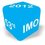# Markovitz portfolio and change of cach system:

Consider a Financial market in which $N$ risky assets are listed. We note $R$ the vector of its return, $\mathcal{E}$ the vector of $R$'s means and $\Lambda$ the matrix of $R$'s covariances. We suppose that $\Lambda$ is not invertible and that the means of returns are not all equals.

Let $P_{\text{min}}$ be the portfolio in which we invest $1$/extract_itex] at $t=0$ and having a minimal variance. We do the following change of cach: instead of calculating the prices of an asset or a portfolio in dollars, we will evaluate the price in numbers of $P_{\text{min}}$. For instance, if an asset costs $1\$ at $t=0$ it costs $10$ in the new cach (we suppose that we have only two dates $t=0$ and $t=0$). We note $R_{\text{min}}$ the vector of assets's returns in the new cach $P_{\text{min}}$, $\mathcal{E}_{\text{min}}$ the vector of $R_{\text{min}}$'s means and $\Lambda_{\text{min}}$ the matrix of $R_{\text{min}}$'s covariances. Explain why the matrix $\Lambda_{\text{min}}$ is not invertible (its rank is $N-1$).Note by Mountassir Farid 5 years, 7 months ago This discussion board is a place to discuss our Daily Challenges and the math and science related to those challenges. Explanations are more than just a solution — they should explain the steps and thinking strategies that you used to obtain the solution. Comments should further the discussion of math and science. When posting on Brilliant: • Use the emojis to react to an explanation, whether you're congratulating a job well done , or just really confused . • Ask specific questions about the challenge or the steps in somebody's explanation. Well-posed questions can add a lot to the discussion, but posting "I don't understand!" doesn't help anyone. • Try to contribute something new to the discussion, whether it is an extension, generalization or other idea related to the challenge. • Stay on topic — we're all here to learn more about math and science, not to hear about your favorite get-rich-quick scheme or current world events. MarkdownAppears as *italics* or _italics_ italics **bold** or __bold__ bold - bulleted- list • bulleted • list 1. numbered2. list 1. numbered 2. list Note: you must add a full line of space before and after lists for them to show up correctly paragraph 1paragraph 2 paragraph 1 paragraph 2 [example link](https://brilliant.org)example link > This is a quote This is a quote  # I indented these lines # 4 spaces, and now they show # up as a code block. print "hello world" # I indented these lines # 4 spaces, and now they show # up as a code block. print "hello world" MathAppears as Remember to wrap math in $$ ... $$ or \[ ... $ to ensure proper formatting.
2 \times 3 $2 \times 3$
2^{34} $2^{34}$
a_{i-1} $a_{i-1}$
\frac{2}{3} $\frac{2}{3}$
\sqrt{2} $\sqrt{2}$
\sum_{i=1}^3 $\sum_{i=1}^3$
\sin \theta $\sin \theta$
\boxed{123} $\boxed{123}$